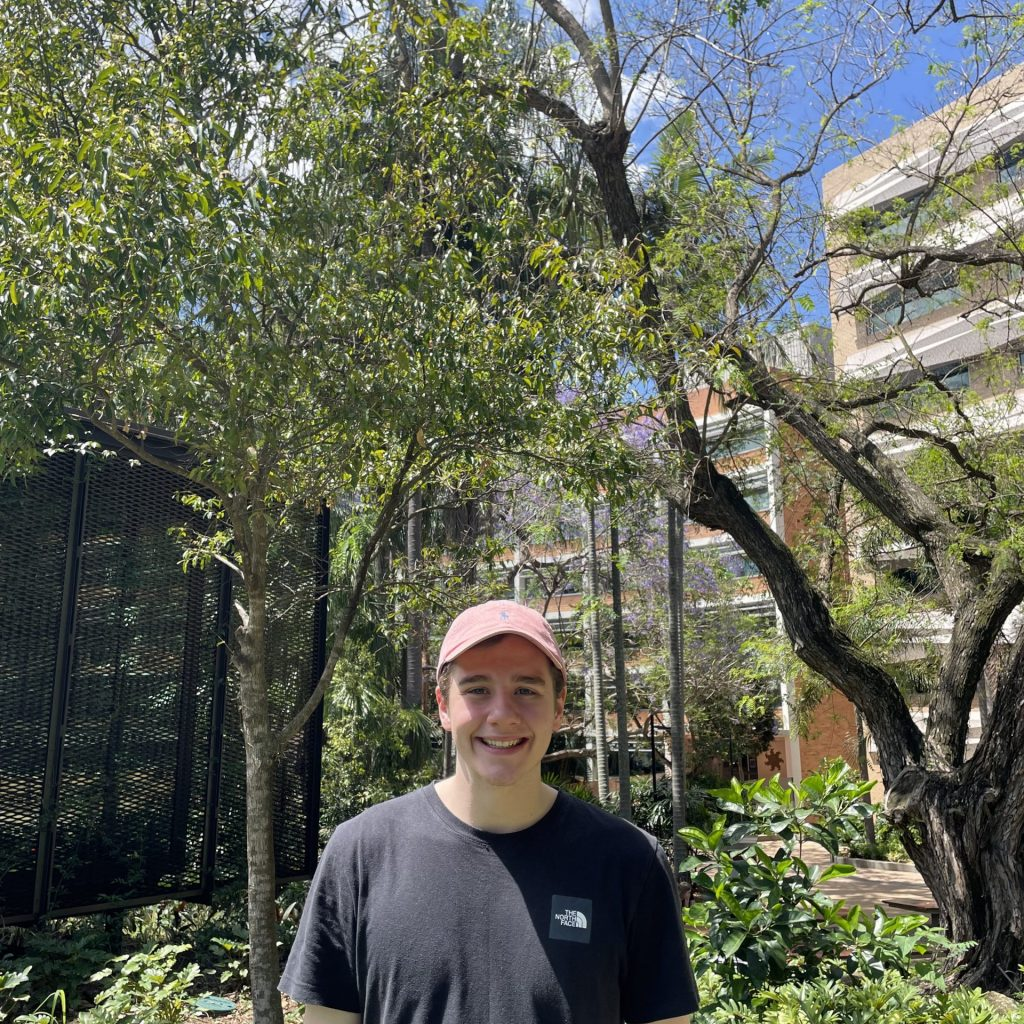An extension to the standard model of particle physics is the theory of supersymmetry. In the current standard model of particle physics, two families of particles, bosons and fermions, are treated separately with commuting and anti-commuting coordinates respectively. Supersymmetry is a spacetime symmetry between the two. For this, it is useful to introduce the idea of a supermanifold, a space that has both commuting and anti-commuting coordinates. This report details the different constructions of a supermanifold and describes connections between them.

A smooth manifold is the generalisation of a surface to higher dimensions; it is a space that locally looks like Euclidean space. One of the aims of supergeometry is to generalise this idea of a manifold to encompass extra coordinates in an attempt to provide supersymmetry and other physical theories with a convenient mathematical groundwork.

Supersymmetry, first introduced in the 1970s, is a theory of particle physics developed to unify the treatment of two types of elementary particles, fermions and bosons. Fermions are matter-carrying particles while bosons are the force carriers in our current standard model of particle physics.

Classically, bosons are dealt with in standard coordinates while fermions anti-commuting coordinates. A prediction made by the theory of supersymmetry is the existence of ‘partner’ particles: for each boson (resp. fermion), there exists a partner fermionic (resp. bosonic) particle. For example, the electron and its partner, the selectron.

The theory of supersymmetry is built upon supergeometric objects, the most foundational of which is a supermanifold. Supermanifolds were defined independently by Batchelor, Berezin, DeWitt, Kostant, Leites, and Rogers.

There exist two main constructions of supermanifolds: the concrete and the algebro-geometric approach. Batchelor, DeWitt and Rogers follow the concrete approach, describing supermanifolds as spaces that locally resemble flat superspace, the model space in supergeometry. In contrast, Berezin, Kostant and Leites describe the algebro-geometric approach, defining a supermanifold as a topological space equipped with a sheaf of Z/2Z-graded algebras. This is analogous to how we can view a classical smooth manifold as a ringed space that is locally isomorphic to Euclidean space with its sheaf of smooth functions.

We deal with the multiple different definitions, understanding and breaking down how they are different from each other as well as where they agree. In the concrete constructions of Batchelor, DeWitt and Rogers, the main differences arise from the difference in the underlying algebra of supernumbers. We can also endow flat superspace (which is built up of subalgebras of supernumbers) with different topologies. DeWitt’s definition uses a very coarse topology while Rogers’ and Batchelor use the product topology, a much finer topology.

On the other hand, the more algebraic approaches all turn out to be equivalent. What is curious, is that the Batchelor supermanifolds are then equivalent to the algebraically defined supermanifolds of Berezin, Kostant and Leites.

Benjamin Kruger
University of QueenslandView Benjamin Kruger
Profile